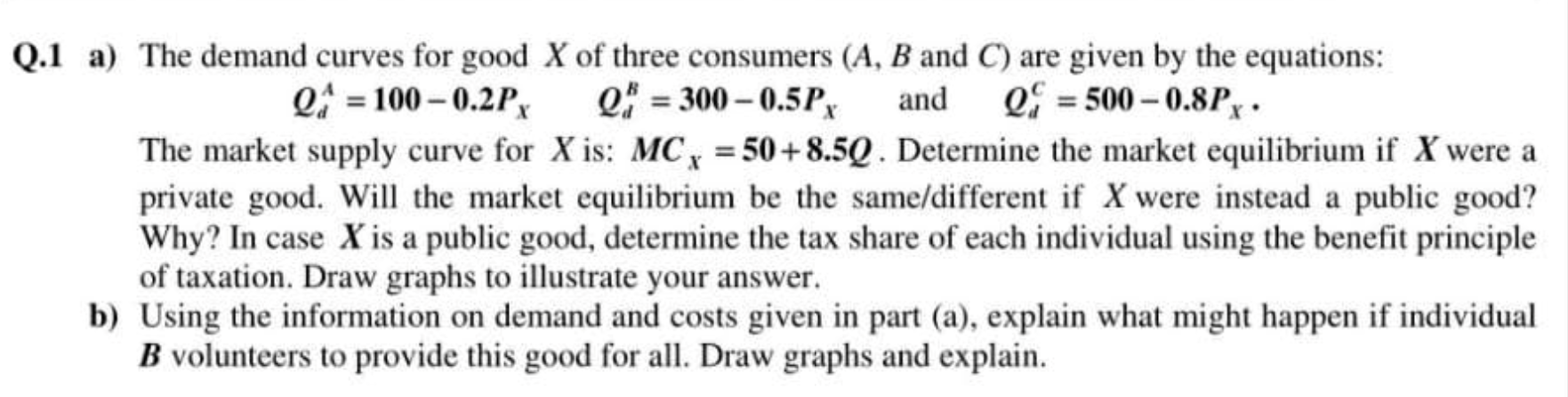### Create an Account

Home / Questions / The demand curves for good X of three consumers (A, B and C) are given by the equations: Q...

# The demand curves for good X of three consumers (A, B and C) are given by the equations: Q = 100 -0.2P Q = 300 - 0.5PX and Oy E 500 -0.8Px. The market supply curve for X is: MC, = 50+ 8.5Q. Determine

The demand curves for good X of three consumers (A, B and C) are given by the equations: Q = 100 -0.2P Q = 300 - 0.5PX and Oy E 500 -0.8Px. The market supply curve for X is: MC, = 50+ 8.5Q. Determine the market equilibrium if X were a private good. Will the market equilibrium be the same/different if X were instead a public good? Why? In case X is a public good, determine the tax share of each individual using the benefit principle of taxation. Draw graphs to illustrate your answer. b) Using the information on demand and costs given in part (a), explain what might happen if individual B volunteers to provide this good for all. Draw graphs and explain.Apr 10 2021 View more View LessSubscribe To Get Solution